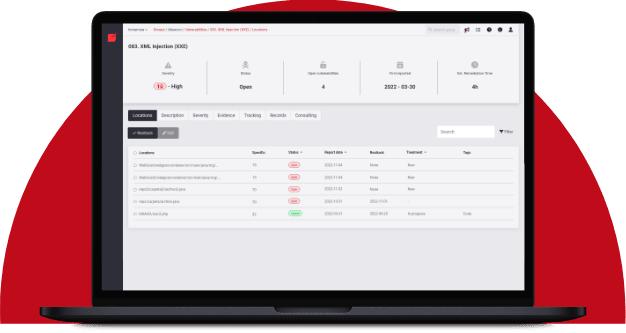# Hit or Miss

## Estimating attack probabilityRafael Ballestas

March 19, 2019

One of the main obstacles against adopting a quantitative approach to risk management is that since major security breaches are relatively rare and hence, there cannot be enough data for proper statistical analysis. While this might be true in the classical sense, it is not if we adopt a Bayesian mindset, which basically amounts to being open to change your beliefs due to new evidence.

Remember the Rule of 5? It allows us to give a 90% confidence interval with only 5 samples. This is already a counterexample for the "not enough data" obstacle. Also recall how we used probability distributions in order to run simulations on many possible scenarios and updated our beliefs based upon evidence, all based only on a few expertly estimated probabilities. In this article we will show how a probability distribution can be derived from simple observations.

Suppose we want to estimate the batting average --the ratio of hits to the number of times he stands at the bat-- for a particular player. One way to do so would be to look at their rolling average, i.e., his average so far. The law of large numbers tells us that no matter what happens at the beginning, the rolling average will tend to the true value, if you observe it for long enough: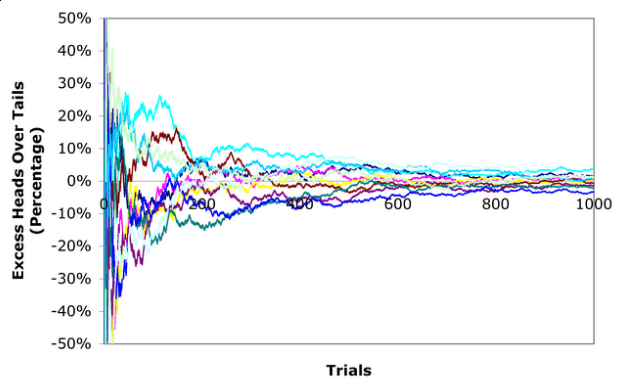Figure 1. Rolling average tends to the true mean. Via Brad DeLong.

The only problem is, we don’t have long enough. Baseball seasons are finite, and major cybersecurity events are few and far between. What is one to do? If we go with the rolling average like in the above image, we would be stuck with the initial, imprecise part of it. For instance, after the first try, the player’s average will be either exactly 0 or 1, which clearly does not reflect the reality well.

Enter the beta probability distribution. This distribution takes two parameters which determine its shape and spread, and are cryptically called alpha and beta, but in reality can be though of as hits and misses from a certain sample. We may also think that the density function of this distribution gives us the probability that a proportion, ratio or probability of an event is just that. No, it was not a typo. We can think of the beta distribution as being the probability distribution of probabilities themselves. As such, we can use it to obtain the probability of being attacked after having observed who has been attacked (the hits) and who has not (the misses) in a certain period of time.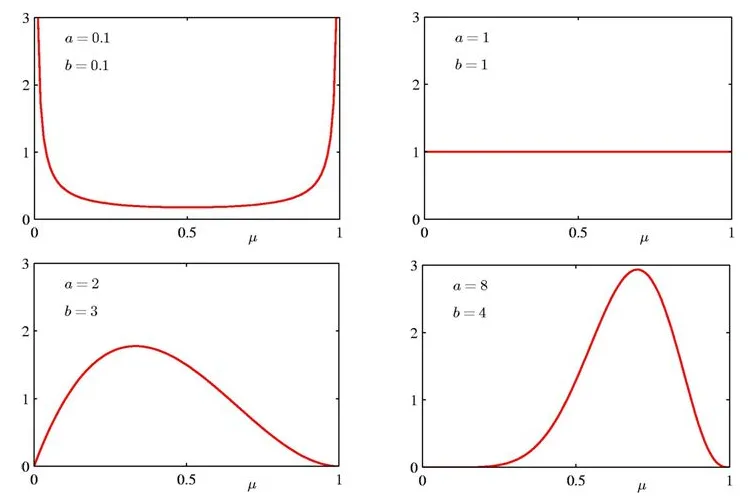Figure 2. Beta distribution with different parameters. By Shona Shields on Slideplayer.

Wait: it gets better. The beta distribution can be updated with evidence and observations, just like we did when working with Bayes Rule, to give better estimations. Since alpha and beta represent hits and misses, and if we observe some breaches and some non-breaches, why not just add them to the original parameters?

It can be shown that the beta distribution, modified this way, reflects reality much better than the previous estimate. And we can continue doing this in a repetitive manner everytime there is an observation.

Imagine an even simpler situation: what is the probability that a coin lands heads? We don’t know whether the coin is fair or has been loaded to give more priority to some results than others, so we might just roll it many times, record the results (how many heads and how many tails) and fit a beta distribution in the manner described above. The results would be as follows: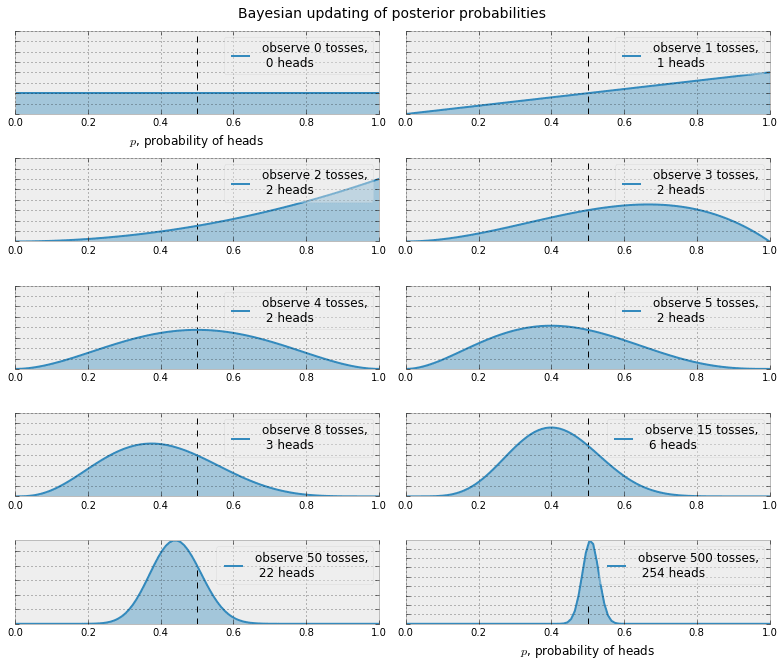Figure 3. Adjusting a beta distribution to new evidence

Notice how the distributions after the first two tosses resulted in head do not just say that the probability of heads is 100%, which is what the rolling average would point to, which is clearly wrong. Instead, the beta distribution sort of smoothes out what would be a sharp, extreme yes/no situation, allowing a chance to values in between. After only 3 tosses the distribution starts to look like a proper distribution. It gives the probability that the probability of obtaining heads has a certain value. After 50 tosses we can conclude, with evidence and a mathematically sound supporting method, that the coin was fair after all.

Next, how do we go about applying this to security breaches? What exactly would be the "hits" and the "misses"? Recall that we update our knowledge of hits and misses by taking random (tough typically small) samples from an unknown, allegedly large population. Since we want to estimate the probability that a business like our own would suffer a major attack, then the population should be a list of companies similar to ours. Call that the top 10, 100, etc of your country/region/world. Out of those, take a random sample, and check against a public database of cybersecurity events (such as the Verizon Data Breach Investigations Report) to see if any of the sampled companies suffered an attack.

We also need seeds for the alpha and beta parameters. These could be expert estimations or, if you want to be very conservative, you can set both to 1, which would give simply a uniform distribution (everything is equally likely). This is the most uninformative of all possible priors. It is totally unbiased. Again, by the law of large numbers, it doesn’t really matter much where we begin. But the better the initial estimates, the faster the convergence to the "truth". Starting with this uniform prior and observing that there is one attacked company in the sample over a 2-year period, we obtain the following beta distribution: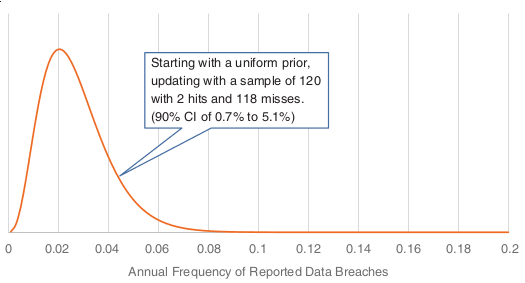Figure 4. Beta distribution for breach frequency.

When we have a distribution, we know pretty much everything. We can give find an expected probability of attack or, better yet, a 90% confidence interval in which that probability lies. We can also use it to update our previous models. Remember that in our simulations to obtain the Loss Exceedance Curve, we used a log-normal distribution simply because it was the best fit due to some of its properties. Now we have a better reason to use this beta distribution we obtained here, and running the simulations again with this distribution would yield the following results: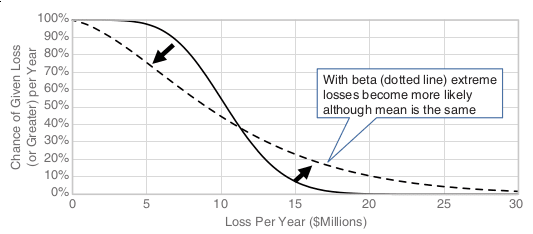Figure 5. Updated LEC

Notice how, by using the beta distribution, it is clear that higher losses are more likely, while smaller losses are less so. Given that this beta distribution was built using real data, this should be a more appropriate estimate of reality.

Thus, the Bayesian interpretation of statistics and, in particular, the iterative updating of a fitted beta distribution can aid your company in better understanding risk, and not only in cibersecurity, since nothing in this method is inherent to cybersecurity risk. Especially in combination with random simulations, which turn these abstract distributions into concrete bills and coins.

## References

1. D. Hubbard, R. Seiersen (2016). How to measure anything in cibersecurity risk. Wiley.

2. M. Richey M. and P. Zorn (2005). Basketball, Beta, and Bayes. Mathematics Magazine, 78(5), 354.

3. D. Robinson (2015). Understanding the beta distribution (using baseball statistics). Variance Explained.

Share

Tags:

## Recommended blog posts

You might be interested in the following related posts.Benefits of continuous over point-in-time pentestingFor which security standards is pentesting a must-have?Pentesting is a system-agnostic approach to securityDifferences between these security testing approachesOur CLI is an approved AST tool to secure cloud appsHow BAS solutions work, their importance and benefitsDisclosure rules proposed by SEC may soon take effectA simple approach to try out in cybersecurity training

## Start your 21-day free trial

Discover benefits of our Continuous Hacking solution, which hundreds of organizations are already enjoying.